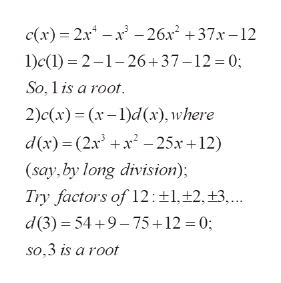Question
1 views

c(x)=2x4-1x3-26x2+37x-12

What are the zeros of c(x)?

check_circle

Step 1

To find the zeros: ( roots of c(x) =0)

Step 2

Find 1 and 3 to be roots , t...help_outlineImage Transcriptionclosec(x) 2x-26x2 +37x-12 1)c() 21-26+37-12=0; So, 1is a root 2)c(x) (x-l)d (x), where d(x) (2xx25x 12) (say,by long division) Try factors of 12: ±1,12, ±3, .. d(3) 54+9-75+12 = 0; | so,3 is a root fullscreen

### Want to see the full answer?

See Solution

#### Want to see this answer and more?

Solutions are written by subject experts who are available 24/7. Questions are typically answered within 1 hour.*

See Solution
*Response times may vary by subject and question.
Tagged in

### Math